## Abstract

A new attractive potential field function is proposed in this paper for manipulator trajectory planning. Existing attractive potential field constructs a global minimum through which maneuvering objects move down the gradient of the potential field toward this global minimum. The proposed method constructs a potential field with two minima. The purpose of these two minima is to create a dual attraction between links rather than affecting each link by the preceding one through kinematic constraints.

## Keywords

Artificial potential field; Articulated robot; Trajectory planning

## 1. Introduction

An Industrial manipulator is a complex nonlinear mechanical system. Large number of degrees of freedom and interaction between manipulator links impose some sort of complexity in constructing direct and inverse dynamic model. A key issue in designing an industrial robot is to simulate the mutual effect of the motion of one link on other links. Essentially, the preceding link motion will change the position of the first joint in the subsequent link. That creates a hierarchy of link (i) over link (i + 1). However, what if link (i + 1) approaches an obstacle? For this circumstance, link (i + 1) should dominate and link (i) has to react accordingly.

Potential field methods provide a simple and elegant solution to many motion planning problems. It works as if a Ferro-material material is placed in a magnetic field, or an electric charge in an electric field. Objects will be attracted to some places in the field while being repelled away from others.

The attraction well is located in such a way it is required to be reached by the maneuvering objects. Both object positions and orientations are considered whenever extended objects are considered. If the maneuvering objects are considered as particles, as considered in majority related papers, positions are only considered in defining the potential field function.

Repulsion zones are defined to protect maneuvering objects from collision with other objects in the workspace. Not only is collision avoidance the role of the repulsive potential, but it also helps in decreasing maneuvering objects kinetic energy when approaching obstacles to smooth their motion, and consequently to reduce the collision avoidance cost.

Variety of applications have emerged for the potential field method during the last quarter century in the fields of robotic manipulators , dynamic obstacles , and moving goal points through defining a potential function that is velocity dependent , and defined over both the Cartesian space and the configuration space . However, limitations of this approach arise when the superposition of the repulsive potential and attractive potential creates local minima. In addition, motion oscillation in the presence of obstacles or in narrow passages and the problem of trapping between two close obstacles also arise . The potential field can then defined as vector functions in  and , which produces a smooth and bounded control and can provide better performance compared to scalar fields. Sliding mode theory (SMC) can be used with the potential field function to perform fast maneuvers .

The rest of this paper is organized as follows. Section 2 discusses attractive potential field function, Section 3 discusses articulated manipulator maneuver strategy, Section 4 presents dual-well potential function, Section 5 presents the numerical results, and finally conclusion of the paper results is presented in Section 6.

## 2. Attractive potential field function

Scalar potential functions are defined to have only one global minimum, to where the maneuvering object is attracted. It moves down-hill through the negative gradient of the potential function until reaching the required goal configuration, at the global minimum. For a point-like object, the potential field can be defined in the form of a parabolic function as follows :

 $V_{att}={\frac {A}{2}}\left(r-r_{g}\right)\cdot \left(r-\right.$$\left.r_{g}\right)+C\left({\overline {q}}\cdot {\overline {q}}\right)$
(1)

where

• A and C are control gains.
• r and rg are the position and goal position of a reference point on the link; for link i, the reference point is joint i.
• ${\textstyle {\overline {q}}}$ is the vector of the error (unit) quaternion ${\textstyle {q_{1}\quad q_{2}\quad q_{3}}^{T}}$. The fourth quaternion parameter will reach its goal value, q4 = 1, as the first three terms reach zero,

 $q_{4}={\sqrt {1-q_{1}^{2}-q_{2}^{2}-q_{3}^{2}}}$
(2)

Minimizing the Euclidian distance between some reference point on the link and its goal position will bring this link to its desired position, whereas attitude is controlled through minimizing the error quaternion. Error quaternion is the difference between the quaternion parameters of the link at any time instant and the goal quaternion parameters. For both quantities, their values should be null at goal configuration. Following Eq. (2), the error quaternion is ${\textstyle {\begin{array}{cccc}0&0&0&1\end{array}}^{T}}$ at goal configuration. Error quaternion is determined as  follows:

 ${\begin{array}{c}q_{1}\\q_{2}\\q_{3}\\q_{4}\end{array}}=\left[{\begin{array}{cccc}q_{4g}&-q_{3g}&q_{2g}&q_{1g}\\q_{3g}&q_{4g}&-q_{1g}&q_{2g}\\-q_{2g}&q_{1g}&q_{4g}&q_{3g}\\-q_{1g}&-q_{2g}&-q_{3g}&q_{4g}\end{array}}\right]{\begin{array}{c}q_{1c}\\q_{2c}\\q_{3c}\\q_{4c}\end{array}}$
(3)

where qg and qc are the goal and current quaternion respectively.

Using the potential function as defined in Eq. (1), the potential forces any maneuvering object to keep moving to minimize the Euclidian distance between its position and the goal position, meanwhile reducing the error quaternion vector so it reaches the required orientation.

## 3. Articulated manipulator maneuver strategy

An articulated industrial robot is composed of several rigid links, such that its first joint is attached to a fixed frame of reference. Consider a robot in 3R configuration as shown in Fig. 1.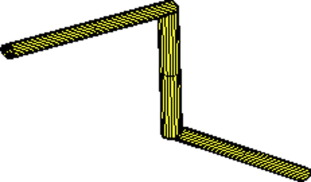Figure 1. Manipulator Architecture.

Since the manipulator links are physically attached, the first point in link (i + 1) has to follow last point in link (i  ). Each link is then described kinematically as a rigid body motion in ${\textstyle R^{3}}$ through the position of a reference point; the first point in each link, and the rotation about this point. This idea is adopted through introducing the position of the attractive well of link (i + 1) at the end of link (i)  as follows:

 $r_{i+1{\mbox{,}}g}={\left[{\begin{array}{cccc}1-2q_{2}^{2}-2q_{3}^{2}&2\left(q_{1}q_{2}-q_{3}q_{4}\right)&2\left(q_{1}q_{3}+q_{2}q_{4}\right)&0\\2\left(q_{1}q_{2}+q_{3}q_{4}\right)&1-2q_{1}^{2}-2q_{3}^{2}&2\left(q_{2}q_{3}-q_{1}q_{4}\right)&0\\2\left(q_{1}q_{3}-q_{2}q_{4}\right)&2\left(q_{2}q_{3}+q_{1}q_{4}\right)&1-2q_{2}^{2}-2q_{1}^{2}&0\\0&0&0&1\end{array}}\right]}_{i}\left[{\begin{array}{c}0\\0\\L_{i}\\1\end{array}}\right]+r_{i}$
(4)

In many cases, the subsequent links suffer zones of high potential due to the existence of obstacles. Consequently, according to the maneuver strategy presented in  only rotational maneuvers are then used for obstacle avoidance. A combination of rotational and translational maneuvers is preferable from the point of view of reducing time and cost .

Considering the case of prismatic joint with its joint parameter in the z direction, the attractive well of link (i + 1) is then related to the motion of link (i) as follows:

 $r_{i+1{\mbox{,}}g}={\left[{\begin{array}{cccc}1-2q_{2}^{2}-2q_{3}^{2}&2\left(q_{1}q_{2}-q_{3}q_{4}\right)&2\left(q_{1}q_{3}+q_{2}q_{4}\right)&0\\2\left(q_{1}q_{2}+q_{3}q_{4}\right)&1-2q_{1}^{2}-2q_{3}^{2}&2\left(q_{2}q_{3}-q_{1}q_{4}\right)&0\\2\left(q_{1}q_{3}-q_{2}q_{4}\right)&2\left(q_{2}q_{3}+q_{1}q_{4}\right)&1-2q_{2}^{2}-2q_{1}^{2}&0\\0&0&0&1\end{array}}\right]}_{i}\left[{\begin{array}{c}0\\0\\L_{i}+d_{i+1}\\1\end{array}}\right]+r_{i}$
(5)

where di+1 is the joint parameter.

## 4. Dual-well potential function

The original method adopted in  with one moving potential well located at the end of the link i will force the first point of link i + 1 to follow the last point in link i whether this motion is suitable or not for link i + 1.

The dual-well potential function provides an interaction of each link with the preceding one. The following link is no longer forced to follow the preceding one nevertheless its own circumstances. The preceding link is required to maneuver to help decrease the overall potential of the whole manipulator. The dual-well attractive potential is defined as

 $V_{att{\mbox{,}}i}={\frac {A}{2}}\left(r_{i}-r_{g{\mbox{,}}i}\right)\cdot \left(r_{i}-\right.$$\left.r_{g{\mbox{,}}i}\right)+{\frac {B}{2}}\left(r_{i+1}-\right.$$\left.r_{g{\mbox{,}}i+1}\right)\cdot \left(r_{i+1}-\right.$$\left.r_{g{\mbox{,}}i+1}\right)+C\left({\overline {q}}_{i}\cdot {\overline {q}}_{i}\right)$
(6)

where A, B, and C are some constants.

The mutual effect of each link on another is achieved since the link (i) attractive potential depends not only on the difference between its own position and its goal position, but also on the difference between the following link and its goal position, which is the end of the preceding link.

In principle, attractive potential functions are defined to ensure convergence to the goal. The most common means of investigating such convergence is using Lyapunov methods that consider convergence to be equivalent to nonlinear stability.

V   is a real, continuously differentiable, positive definite scalar function that maps ${\textstyle R^{n}\rightarrow R}$. Then if its time derivative ${\textstyle {\overset {\cdot }{V}}}$ is negative definite, then V is asymptotically stable  and .

The time derivative of the proposed function will be

 ${\overset {\cdot }{V}}_{att{\mbox{,}}i}=A\left(r_{i}-r_{g{\mbox{,}}i}\right)\cdot \left({\overset {\cdot }{r}}_{i}-\right.$$\left.{\overset {\cdot }{r}}_{g{\mbox{,}}i}\right)+B\left(r_{i+1}-\right.$$\left.r_{g{\mbox{,}}i+1}\right)\cdot \left({\overset {\cdot }{r}}_{i+1}-\right.$$\left.{\overset {\cdot }{r}}_{g{\mbox{,}}i+1}\right)+$$2C\left({\overline {q}}_{i}\cdot {\overset {\cdot }{\overline {q}}}_{i}\right)$
(7)

To satisfy Lyapunov’s theorem, the control laws are then defined as

 ${\overset {\cdot }{r}}_{i}=-k{\frac {\left(r_{i}-r_{g{\mbox{,}}i}\right)}{\left\|\nabla V\right\|}}+$${\overset {\cdot }{r}}_{g{\mbox{,}}i}-\left(r_{i+1}-r_{g{\mbox{,}}i+1}\right)\cdot \left({\overset {\cdot }{r}}_{i+1}-\right.$$\left.{\overset {\cdot }{r}}_{g{\mbox{,}}i+1}\right)$
(8)
 ${\overset {\cdot }{\overline {q}}}=-k_{q}{\frac {\overline {q}}{\left|{\nabla }^{q}V_{att{\mbox{,}}i}\right|}}$
(9)

where

 $k=v_{\mbox{max}}\left(1-e^{-\alpha V_{att{\mbox{,}}i}}\right)$
(10)
 $k_{q}={\omega }_{\mbox{max}}\left(1-e^{-\beta V_{att{\mbox{,}}i}}\right)$
(11)
 ${\nabla }^{q}={\begin{array}{ccc}\partial /\partial q_{1}&\partial /\partial q_{2}&\partial /\partial q_{3}\end{array}}^{T}$
(12)

vmax is the maximum controlled velocity of the manipulator tip. The value of vmax does not have influence on controller decision, but has to be chosen great enough to ensure robust follow of the first joint in the subsequent link to the last joint in the preceding one. Since only revolute joints are considered herein, no control intervention is directly based on the choice of vmax or joint velocities derived from Eq. (8). However, these velocities change the joint position, affect the overall potential function, and consequently affect controller rotational decision. α, β are constants that control the level of attraction wells, and rates of decreasing speeds when approaching goals.

ωmax is the maximum controlled angular velocity of links. It is directly dependent upon actuator available torque and link dynamical properties.

## 5. Numerical results

A simulation of the proposed method is implemented in modeling the motion of manipulator shown in Fig. 1, using Matlab software. The manipulator is required to move from some initial configuration to the final one in 200 s, and the maximum angular velocity for all motors is 0.01 rad/s. All control gains are set to unit. The maneuvering motion of the manipulator is shown in Fig. 2.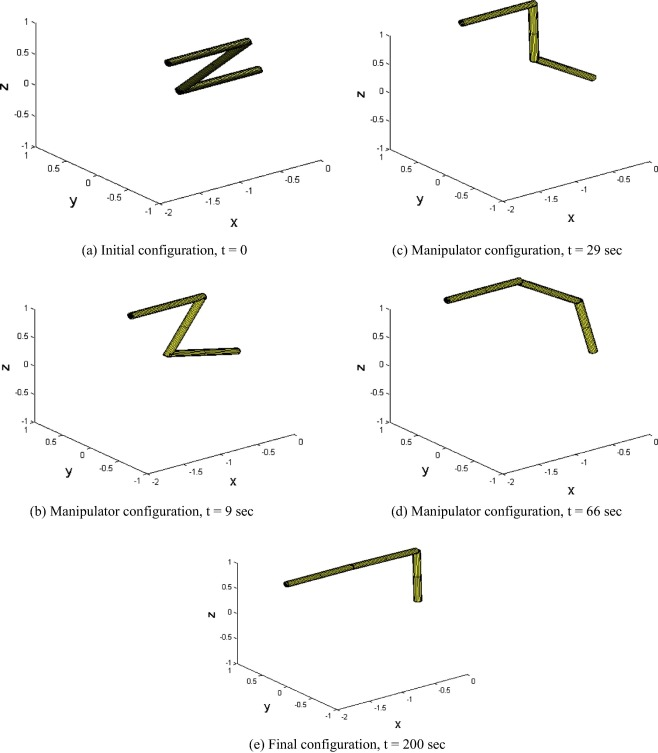Figure 2. Maneuver of three-link manipulator (case I). (a) Initial configuration, t = 0. (b) Manipulator configuration, t = 9 s. (c) Manipulator configuration, t = 29 s. (d) Manipulator configuration, t = 66 s. (e) Final configuration, t = 200 s.

Since the basic link motions are rotation about y-axis, error quaternion parameters about y-axis are shown in Fig. 3. For the three links, error quaternion about y-axis approaches zero asymptotically. Consequently, smooth stop at final orientation of all links is attained.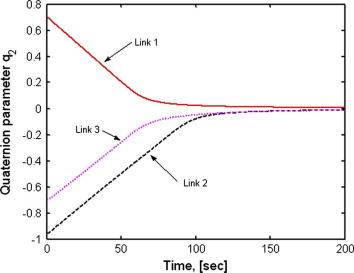Figure 3. Rotation about y-axis.

The simulation is conducted using both the original method  and the proposed method in the absence of obstacles. The proposed method shows faster maneuver despite no obstacles exist; the difference is shown in Fig. 4 for link (1).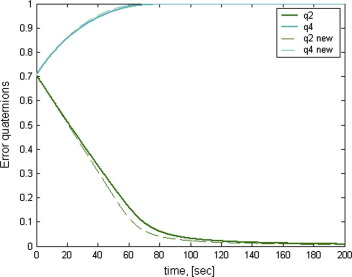Figure 4. Comparison between error quaternion of the first link for both original and dual-well potential function.

A second simulation results for a case of 3D reconfiguration of a three-link manipulator. Keeping all controls constant as for case I, while starting and goal configurations are changed. This simulation shows that there are no limitations for 2D maneuver as in case I. Fig. 5 shows the variation of link configuration at different time steps.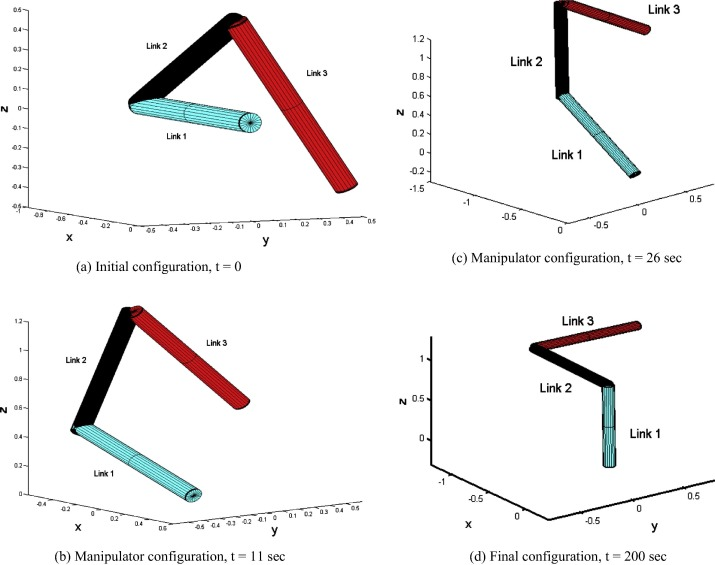Figure 5. Maneuver of three-link manipulator (case II). (a) Initial configuration, t = 0. (b) Manipulator configuration, t = 11 s. (c) Manipulator configuration, t = 26 s. (d) Final configuration, t = 200 s.

A third hypothetical example, similar to case II but with different starting configurations, is discussed with similar control gains as previous cases. Link no. 3 is asked to perform rotations about both x and y-axes. Consequently, the third joint has two rotational degrees of freedom. This type of joint is sometime found at the wrist position of the manipulator, but with zero length links. Fig. 6 shows the starting configuration of the manipulator, while Fig. 7 gives the rotational maneuver as quaternion parameters verses time.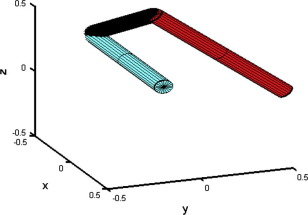Figure 6. Starting configuration of manipulator (case III).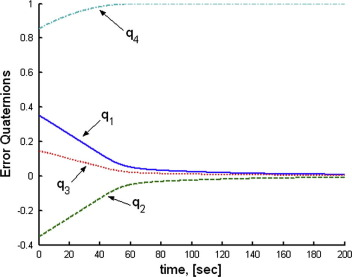Figure 7. Rotation maneuvers of the third link.

In order to demonstrate the power of the current method in trajectory planning of an industrial manipulator, a forth simulation performed with joint (1) has two different types of joint parameters: rotation and translations. This is the case of having a mobile base supporting the manipulator. The base is asked to move 2 [m] in the positive x-direction meanwhile the manipulator is performing rotational maneuvers through its three revolute joints. Motion of the first point in each link is demonstrated in Fig. 8. Rotational maneuver in form of error quaternion is shown in Fig. 9.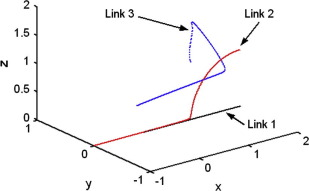Figure 8. Translation of first joint in each link.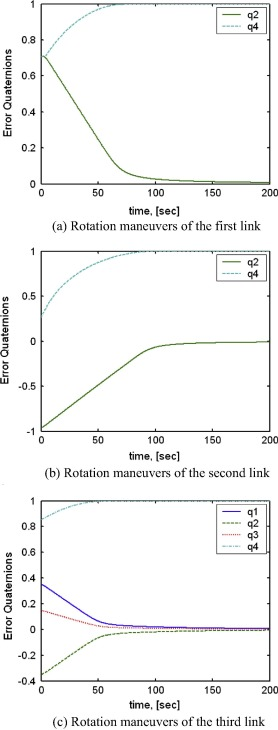Figure 9. Rotation maneuvers of a manipulator with base motion. (a) Rotation maneuvers of the first link. (b) Rotation maneuvers of the second link. (c) Rotation maneuvers of the third link.

The proposed attractive potentials tested for faster manipulator using the maximum angular velocity for all motors are 0.05, 0.1, and 1 rad/s. The results expressed as error quaternion of the first link are shown in Fig. 10. The dependency of the maneuvering rate is apparent as compared to the same case described earlier in Fig. 9a.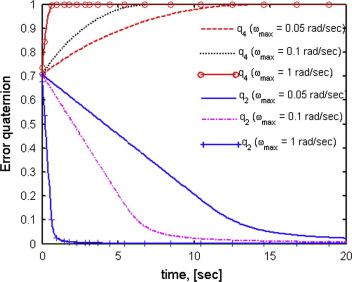Figure 10. Faster rotation maneuvering rates of the first link.

In order to study the effect of control gains that affects both the level of attraction wells, and rates of decreasing speeds when approaching goals, different values of the constant β are examined. The maximum controlled angular velocity is considered as 0.1 rad/s, while β ranges from 0.01 to 1. The results are shown in Fig. 11.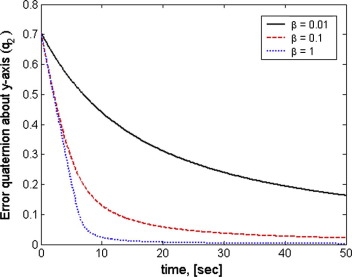Figure 11. Rotational maneuver change verses the rate of decreasing rotation speed at goal configuration.

## 6. Conclusions

The proposed dual-well attractive potential field function for each link is able to solve for trajectory planning of articulated industrial manipulator. The method gives mutual effect between successive links rather than having hierarchy of the preceding link over the following one. Simulations give satisfactory trajectory function since no hasty change in trajectory function is produced. The proposed method gives better results compared to the original potential field method since faster maneuvers are obtained using the same control parameters. The use of quaternion provides no singularities unlike the case of using Euler angles for attitude control. The proposed method handles out different types of joints with single or multi-degrees of freedom. Both revolute and prismatic joints, joints with two degrees of freedom, and even joints with revolute and prismatic degrees of freedom are solved efficiently without major change in the algorithm. Hence, less computational effort is required and consequently less computational capabilities.

### Document informationPublished on 12/04/17

Licence: Other

### Document Score0

Views 14
Recommendations 0